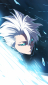# Can you help me po to solve this?

2 posts / 0 new
dragonicewielderCan you help me po to solve this?

A motor A delivers 3000 hp to the shaft at 1500 rev/min of which 1000 hp is removed by gear B and 2000 hp is removed by gear C. Determine (a) the maximum shear stress in the shaft; and (b) the angle of twist of end D relative to end A. Use G=12×10^6 psi for steel, and assume that friction at bearing D is negligible.
a.) maximum shear stress in the shaft, maximum shear stress.
b.) angle of twist of end 'D' relative to end of A, (Theta D/A)

Joselito Cordero Jr (guest)

a.) The maximum shear stress in the shaft can be determined using the formula:

Tmax = P/(2πr^2)

where Tmax is the maximum shear stress, P is the power transmitted by the shaft, and r is the radius of the shaft.

Substituting the given values, we get:

Tmax = 3000 hp / (2π(0.5 in)^2) = 104493.9 psi

b.) The angle of twist of end D relative to end A can be determined using the formula:

Theta D/A = T * L / (G*J)

where T is the maximum shear stress, L is the length of the shaft, G is the shear modulus of the material, and J is the polar moment of inertia of the shaft.

Substituting the given values, we get:

Theta D/A = 104493.9 psi * 1 ft / (12*10^6 psi * (0.5 in)^4) = 0.0035 radians = 0.2 degrees

• Mathematics inside the configured delimiters is rendered by MathJax. The default math delimiters are $$...$$ and $...$ for displayed mathematics, and $...$ and $...$ for in-line mathematics.